## MTSO April News - We are here for you.We hope you are all healthy and doing well. From our family here at MTSO to your family we want you to know that we are in this together, be kind, be helpful to others and stay positive. We have helpful suggestions and ideas that will give you inspiration and energy!

1) We are looking for young talent for our Cabin Fever Art Exhibit
2) A great product for boosting your Immune System
3) Ideas, activities, and suggestions to help others
and much more . . .

 table div table+table+table+table div table{width:100%;padding:0}table div table+table+table+table div table img{width:96.23%;padding:0;float:none}table div table+table+table+table div table td{width:100%;padding:0 1.88% 18px}/* styles */# Ideas for helping others:

1) Call family & friends to check on how they are doing. A simple phone call can make a big difference.
2) Offer to walk dogs, run errands, and check in on neighbors in a high-risk group.
3) Donate to local food banks, women’s shelters and homeless shelters.
4) Donate gift cards, Any charity that takes care of people or animals is going to be very grateful to have gift cards. If you send a gift card, then they can buy whatever they want with it.
5) Surprise a friend or neighbor by leaving flowers or a treat on their doorstep.
6) Be extra nice and kind to everyone you meet.

 table div table+table+table+table+table+table div table{width:100%;padding:0}table div table+table+table+table+table+table div table img{width:96.23%;padding:0;float:none}table div table+table+table+table+table+table div table td{width:100%;padding:0 1.88% 18px}/* styles */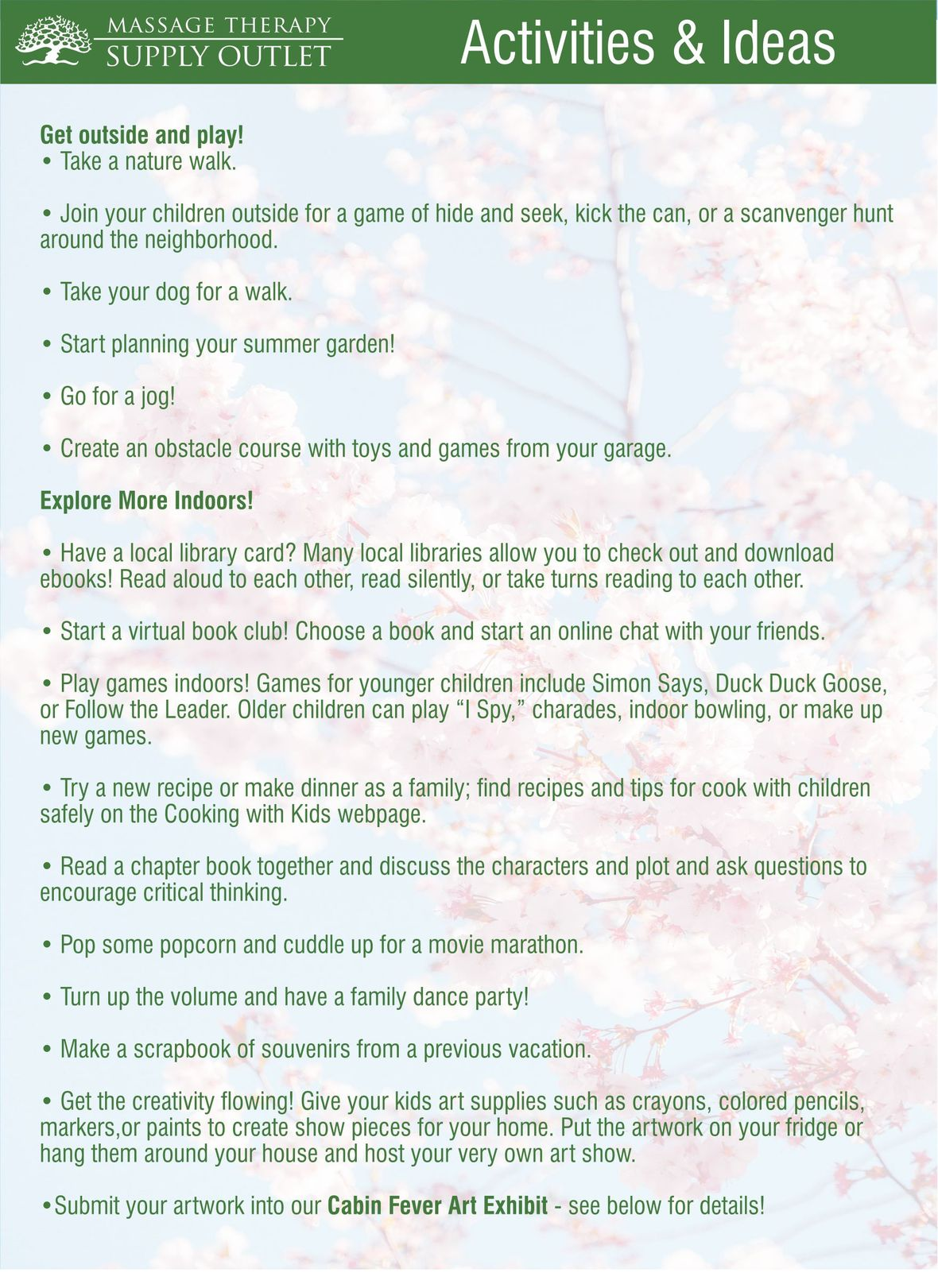table div table+table+table+table+table+table+table+table div table{width:100%;padding:0}table div table+table+table+table+table+table+table+table div table img{width:96.23%;padding:0;float:none}table div table+table+table+table+table+table+table+table div table td{width:100%;padding:0 1.88% 18px}/* styles */# Did You Know?

Massage Therapy Supply Outlet has an ART GALLERY? Gallery U.

MTSO Owner Joe Clare opened the Gallery U for regular exhibitions, which are viewable 24 hours a day.

 table.module-10{width:75.28%;padding:0}table div table+table+table+table+table+table+table+table+table+table+table div table{width:75.28%;float:none;margin-left:auto;margin-right:auto;padding:0}table div table+table+table+table+table+table+table+table+table+table+table div table a{border:0 none;text-decoration:none}table div table+table+table+table+table+table+table+table+table+table+table div table img{width:100%!important;border:0 none;text-decoration:none}table div table+table+table+table+table+table+table+table+table+table+table div table td{width:100%;padding:0}/* styles */
 table div table+table+table+table+table+table+table+table+table+table+table+table div table{width:100%;padding:0}table div table+table+table+table+table+table+table+table+table+table+table+table div table img{width:96.23%;padding:0;float:none}table div table+table+table+table+table+table+table+table+table+table+table+table div table td{width:100%;padding:0 1.88% 18px}/* styles */table.module-12{width:88.3%;padding:0}table div table+table+table+table+table+table+table+table+table+table+table+table+table div table{width:88.3%;float:none;margin-left:auto;margin-right:auto;padding:0}table div table+table+table+table+table+table+table+table+table+table+table+table+table div table a{border:0 none;text-decoration:none}table div table+table+table+table+table+table+table+table+table+table+table+table+table div table img{width:100%!important;border:0 none;text-decoration:none}table div table+table+table+table+table+table+table+table+table+table+table+table+table div table td{width:100%;padding:0}/* styles */
 /* styles */ Getting Cabin Fever? Want inspiration? We have a fun challenge for you. We would like for you to have your children, grandchildren, customer’s children or any children in your life to start creating artwork to be featured in our art exhibit.
 table div table+table+table+table+table+table+table+table+table+table+table+table+table+table+table+table div table{width:100%;padding:0}table div table+table+table+table+table+table+table+table+table+table+table+table+table+table+table+table div table img{width:96.23%;padding:0;float:none}table div table+table+table+table+table+table+table+table+table+table+table+table+table+table+table+table div table td{width:100%;padding:0 1.88% 18px}/* styles */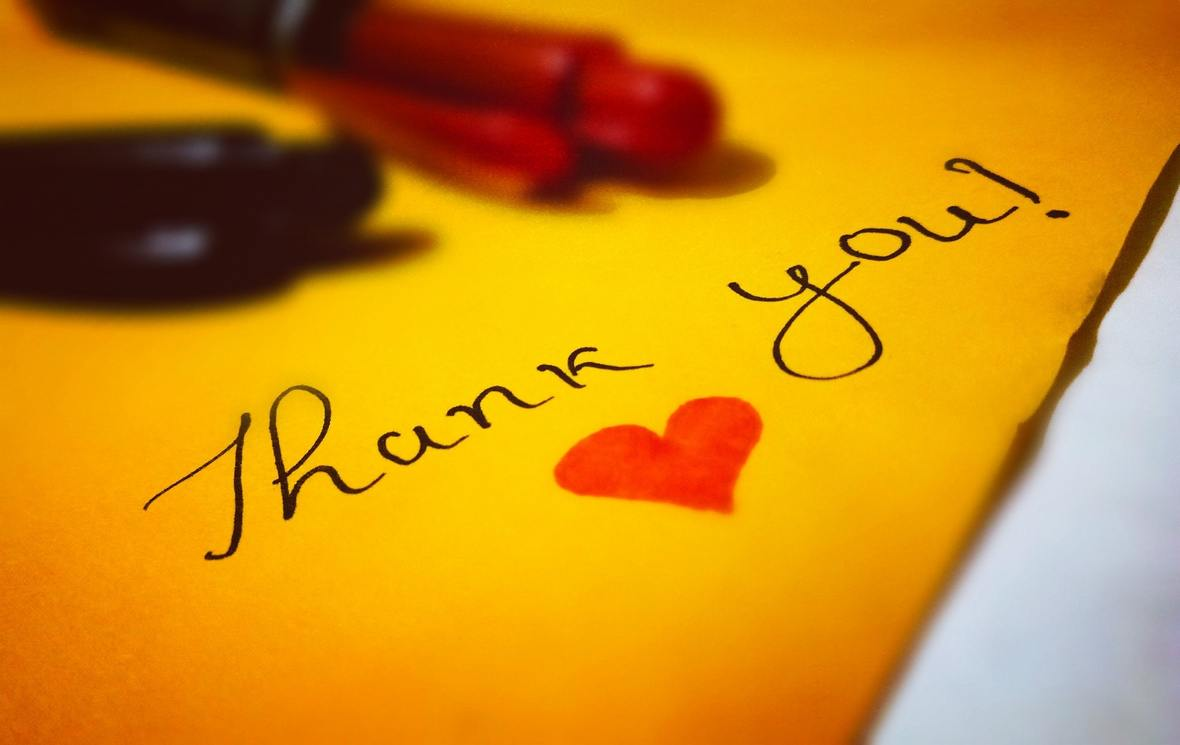/* styles */ The MTSO Family would like to take this time and say THANK YOU to all our customers, family and friends. We appreciate you for the support and inspiration you have given us. This is also a time for all of us to say THANK YOU and recognize the people in our community. Show your appreciation in making a sign or another form of communication to share your message of thankfulness to people or groups in your area.
 table div table+table+table+table+table+table+table+table+table+table+table+table+table+table+table+table+table+table+table div table{width:100%;padding:0}table div table+table+table+table+table+table+table+table+table+table+table+table+table+table+table+table+table+table+table div table img{width:96.23%;padding:0;float:none}table div table+table+table+table+table+table+table+table+table+table+table+table+table+table+table+table+table+table+table div table td{width:100%;padding:0 1.88% 18px}/* styles */# MYSHROOMS IMMUNITY (MYSPRAY)

MyShrooms Immunity offers the synergistic effects of eight medicinal mushrooms, each containing complex, unique and specific compounds providing significant health benefits throughout the whole body as a daily immune modulator. 8 mushrooms in a chocolate liquid base, consumed by spraying under the tongue.

 table div table+table+table+table+table+table+table+table+table+table+table+table+table+table+table+table+table+table+table+table+table+table div table{width:100%;padding:0}table div table+table+table+table+table+table+table+table+table+table+table+table+table+table+table+table+table+table+table+table+table+table div table img{width:96.23%;padding:0;float:none}table div table+table+table+table+table+table+table+table+table+table+table+table+table+table+table+table+table+table+table+table+table+table div table td{width:100%;padding:0 1.88% 18px}/* styles */# Towel Warmer Standard 120V UV

## SALE PRICE: \$325.00

Reg Price: \$350.00
This dependable UL listed unit heats and sanitizes approximately 24 towels; it offers a sleek design featuring stainless steel interior, and a discrete drip pan. This unit offers a compact footprint design, that heats and sanitizes your cloth or disposable towels for continuous use throughout the day.

 table div table+table+table+table+table+table+table+table+table+table+table+table+table+table+table+table+table+table+table+table+table+table+table+table+table div table{width:100%;padding:0}table div table+table+table+table+table+table+table+table+table+table+table+table+table+table+table+table+table+table+table+table+table+table+table+table+table div table img{width:96.23%;padding:0;float:none}table div table+table+table+table+table+table+table+table+table+table+table+table+table+table+table+table+table+table+table+table+table+table+table+table+table div table td{width:100%;padding:0 1.88% 18px}/* styles */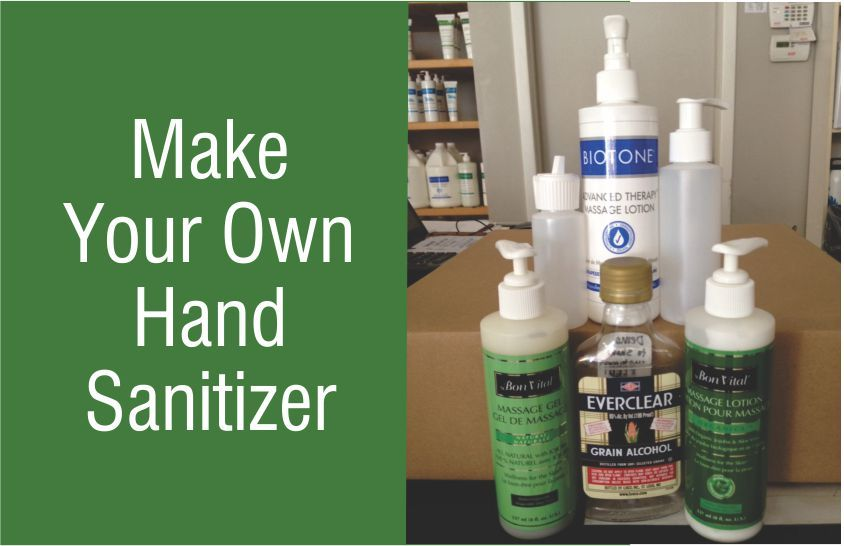# HOW TO MAKE YOUR OWN HAND SANITIZER

Dilute any massage gel or lotion by half (50%) with Everclear Grain Alcohol 95% alcohol.
Pour into your own squeeze or pump bottle & use as needed.
70% Isopropyl rubbing alcohol can be used in a slightly higher concentration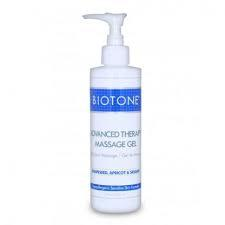BIOTONE ADVANCED THERAPY MASSAGE GEL 8OZ PUMP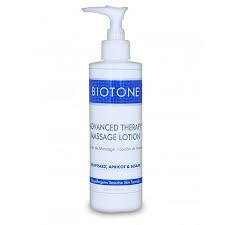BIOTONE ADVANCED THERAPY MASSAGE LOTION 8OZ PUMP
 table div table+table+table+table+table+table+table+table+table+table+table+table+table+table+table+table+table+table+table+table+table+table+table+table+table+table+table+table+table div table{width:100%;padding:0}table div table+table+table+table+table+table+table+table+table+table+table+table+table+table+table+table+table+table+table+table+table+table+table+table+table+table+table+table+table div table img{width:96.23%;padding:0;float:none}table div table+table+table+table+table+table+table+table+table+table+table+table+table+table+table+table+table+table+table+table+table+table+table+table+table+table+table+table+table div table td{width:100%;padding:0 1.88% 18px}/* styles */ATTENTION TO ALL OF OUR DEAR CUSTOMERS:

Please note due to the importance of minimizing social contact, MTSO is recommending all local and out of town customers place their orders online. We can arrange delivery through our courier service and kindly visit the shipping department at the back door of the store underneath the stairs for pick up between 12pm-4pm. Please call us at 780-440-1818 when you arrive in the parking area of our store to pick up your order.

We appreciate your understanding and wishing you good health.

-The Staff at Massage Therapy Supply Outlet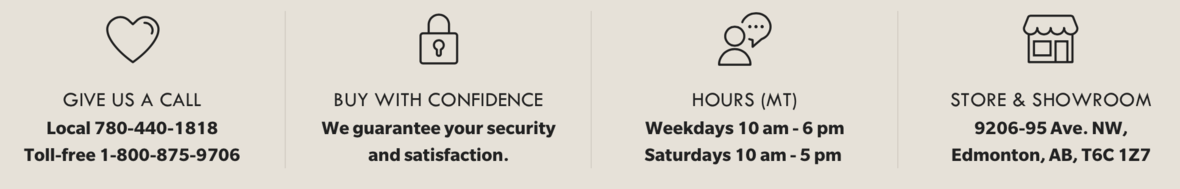## Online Store only available at this time

Browse and buy from an extensive collection at our online store, always open, 24/7/365. We can arrange delivery through our courier service and kindly visit the shipping department at the back door of the store underneath the stairs for pick up between 12pm-4pm. Please call us at 780-440-1818 when you arrive in the parking area of our store to pick up your order.

 table.module-32{width:51.32%;padding:0}table div table+table+table+table+table+table+table+table+table+table+table+table+table+table+table+table+table+table+table+table+table+table+table+table+table+table+table+table+table+table+table+table+table div table{width:51.32%;float:none;margin-left:auto;margin-right:auto;padding:0}table div table+table+table+table+table+table+table+table+table+table+table+table+table+table+table+table+table+table+table+table+table+table+table+table+table+table+table+table+table+table+table+table+table div table a{border:0 none;text-decoration:none}table div table+table+table+table+table+table+table+table+table+table+table+table+table+table+table+table+table+table+table+table+table+table+table+table+table+table+table+table+table+table+table+table+table div table img{width:100%!important;border:0 none;text-decoration:none}table div table+table+table+table+table+table+table+table+table+table+table+table+table+table+table+table+table+table+table+table+table+table+table+table+table+table+table+table+table+table+table+table+table div table td{width:100%;padding:0}/* styles */
 Like   Tweet   Pin   in